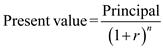# Quiz 1: Managers, Profits, and Markets

Business

There is no answer for this question

Given information Compute Future value of \$1,000 for three different time period at a discount rate of 6.5%.Where, r = discount rate and n = time period a. For a time period of one year, the present value of \$1,000 would be:Thus, Present value is equal to \$938.97. b. A payment of \$1,000 which would be received at the end of two years would have a present value equal to:Thus, Present value is equal to \$881.66. c. A payment of \$1,000 which would be received at the end of three years would have a present value equal to:Thus, Present value is equal to \$827.85.

An audio engineer started his own business leaving his job where he was earning a salary of \$175,000 per year. And he spent his savings to start his business which could earn an interest of \$15,000. a. The following point explains various cost and income during his business: Explicit cost refers to the cost of using outside resources. Examples of such costs are wage, salaries, rents and taxes. Therefore explicit cost would be:Thus, explicit for first year is \$793,000. Implicit cost refers to the opportunity cost of choosing an option. In this case engineer left his job and put his personal saving of \$100,000 that could have earned an interest of \$15,000 a year.Thus, implicit cost is \$190,000. Total economic cost is total of explicit and implicit costs. It can be calculate in following manner:Thus, economic cost is \$983,000. b. Accounting profit is the difference between total revenue and explicit cost. Here opportunity cost is not considered. It can be calculate in following manner:Thus, accounting profit is \$177,000. c. Economic profit is the difference between total revenue and total cost which includes both explicit and implicit costs. It can be calculate in following manner:Thus, first year this business earned an economic loss of \$13,000. d. The economic profit for SD Inc. is in negative which means an economic loss in the first year. But there is an accounting profit in the first year. Therefore, there could be an expected growth and increase in profits in the further years as the cost reduces and income increases. A judgment cannot be passed on the basis of one year profit and loss result.

There is no answer for this question